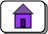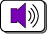created: 05/27/2002; revisions:11/06/02, 11/26/03; 05/24/2015, 08/01/2020CHAPTER 29 — Binary Fractions

Up until now all of the arithmetic we have done on the MIPS has been with integers, both signed (two's complement) and unsigned. This chapter starts the discussion of how floating point arithmetic is done on modern computers (including MIPS.)

Chapter Topics:

• Positional notation with fractions
• Converting fractions between base two and base ten
• Fixed point representation
• Limited precision of binary fractions (fixed point and floating point)
• How "one tenth" cannot be expressed in binary
• Dangerous program loops

QUESTION 1:

Is is possible to represent a negative integer with a positive number of bits?